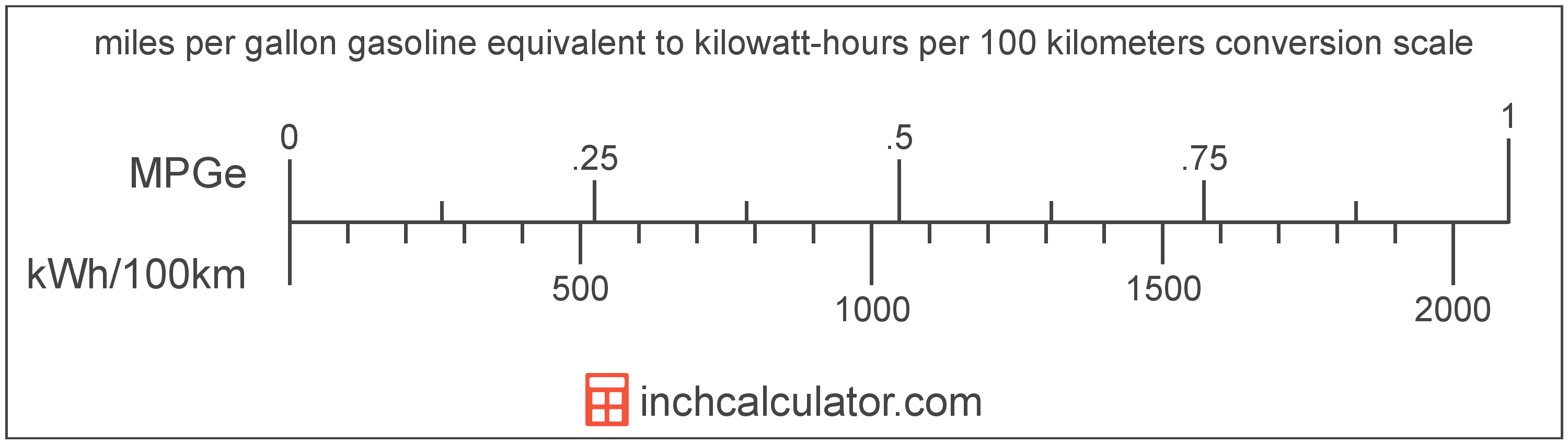# Miles per Gallon Gasoline Equivalent to Kilowatt-Hours per 100 Kilometers Converter (MPGe to KWh/100km)

Enter the electric car efficiency in miles per gallon gasoline equivalent below to get the value converted to kilowatt-hours per 100 kilometers.

Results in Kilowatt-Hours per 100 Kilometers:1 MPGe = 2,094.331603 kWh/100km

Do you want to convert kWh/100km to MPGe?

## How to Convert Miles per Gallon Gasoline Equivalent to Kilowatt-Hours per 100 Kilometers

Convert miles per gallon gasoline equivalent to kilowatt-hours per 100 kilometers with this simple formula:

kWh/100km = 3370.5 ÷ 1.609344 ÷ MPGe

Insert the MPGe efficiency measurement in the formula and then solve to find the result.

For example, let's convert 50 MPGe to kWh/100km:

50 MPGe = ( 3370.5 ÷ 1.609344 ÷ 50 ) = 41.886632 kWh/100kmMiles per gallon gasoline equivalent and kilowatt-hours per 100 kilometers are both units used to measure electric car efficiency. Keep reading to learn more about each unit of measure.

## What Are Miles per Gallon Gasoline Equivalent?

Miles per gallon gasoline equivalent is a way of measuring the energy needed equivalent to a gallon of gasoline to travel a distance in miles.

Because electric vehicles do not consume gasoline, the EPA set an equivalent amount of energy in kilowatt-hours that is equal to one gallon of gasoline. According to the EPA, one gallon of gasoline is equal to 33.705 kilowatt-hours.

The mile per gallon gasoline equivalent is a US customary unit of electric car efficiency. Miles per gallon gasoline equivalent can be abbreviated as MPGe; for example, 1 mile per gallon gasoline equivalent can be written as 1 MPGe.

## What Are Kilowatt-Hours per 100 Kilometers?

Kilowatt-hours per 100 kilometers are a measure of electric vehicle energy efficiency equal to the kilowatt-hours of energy needed for a vehicle to travel 100 kilometers.

The kilowatt-hour per 100 kilometers is an SI unit of electric car efficiency in the metric system. Kilowatt-hours per 100 kilometers can be abbreviated as kWh/100km; for example, 1 kilowatt-hour per 100 kilometers can be written as 1 kWh/100km.

In the expressions of units, the slash, or solidus (/), is used to express a change in one or more units relative to a change in one or more other units.

## Mile per Gallon Gasoline Equivalent to Kilowatt-Hour per 100 Kilometers Conversion Table

Table showing various mile per gallon gasoline equivalent measurements converted to kilowatt-hours per 100 kilometers.
Miles Per Gallon Gasoline Equivalent Kilowatt-hours Per 100 Kilometers
0.001 MPGe 2,094,332 kWh/100km
0.002 MPGe 1,047,166 kWh/100km
0.003 MPGe 698,111 kWh/100km
0.004 MPGe 523,583 kWh/100km
0.005 MPGe 418,866 kWh/100km
0.006 MPGe 349,055 kWh/100km
0.007 MPGe 299,190 kWh/100km
0.008 MPGe 261,791 kWh/100km
0.009 MPGe 232,704 kWh/100km
0.01 MPGe 209,433 kWh/100km
0.02 MPGe 104,717 kWh/100km
0.03 MPGe 69,811 kWh/100km
0.04 MPGe 52,358 kWh/100km
0.05 MPGe 41,887 kWh/100km
0.06 MPGe 34,906 kWh/100km
0.07 MPGe 29,919 kWh/100km
0.08 MPGe 26,179 kWh/100km
0.09 MPGe 23,270 kWh/100km
0.1 MPGe 20,943 kWh/100km
0.2 MPGe 10,472 kWh/100km
0.3 MPGe 6,981 kWh/100km
0.4 MPGe 5,236 kWh/100km
0.5 MPGe 4,189 kWh/100km
0.6 MPGe 3,491 kWh/100km
0.7 MPGe 2,992 kWh/100km
0.8 MPGe 2,618 kWh/100km
0.9 MPGe 2,327 kWh/100km
1 MPGe 2,094 kWh/100km

## References

1. United States Environmental Protection Agency, Technology, https://www3.epa.gov/otaq/gvg/learn-more-technology.htm